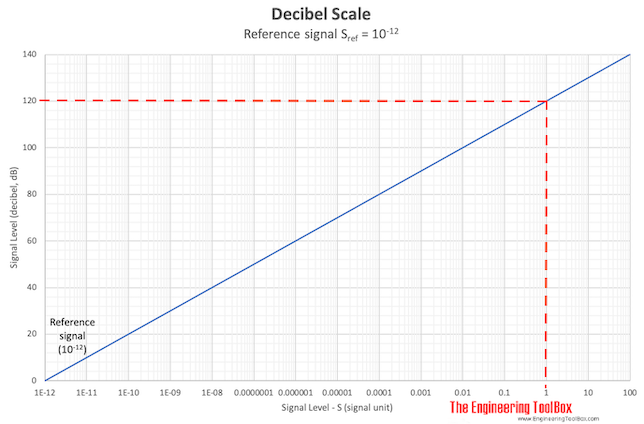Engineering ToolBox - Resources, Tools and Basic Information for Engineering and Design of Technical Applications!

# Decibel

## Logarithmic unit used to describe ratios of signal levels - like power or intensity - to a reference level.

The decibel is a logarithmic unit used to describe the ratio of a signal level - like power or intensity - to a reference level.

• the decibel express the level of a value relative to a reference value

### Decibel Definition

The decibel level of a signal can be expressed as

L = 10 log (S / Sref)                                                       (1)

where

L = signal level (decibel, dB)

S = signal - intensity or power level (signal unit)

Sref = reference signal - intensity or power level (signal unit)

Decibel is a dimensionless value of relative ratios. The signal units depends on the nature of the signal - can be W for power.

A decibel is one-tenth of a Bel - named after Alexander Graham Bell, the inventor of the telephone.

Note! - the decibel value of a signal increases with 3 dB if the signal is doubled (L = 10 log (2) = 3).

If the decibel value and reference level are known the absolute signal level can be calculated by transforming (1) to

S = Sref 10(L / 10)                                                      (2)

### Decibel Calculator

S - signal - intensity or power level (signal unit)

Sref - reference signal - intensity or power level (signal unit)

### Example - Lowest Hearable Sound Power

10-12 W is normally the lowest sound power possible to hear and this value is normally used as the reference power in sound power calculations.

The sound power in decibel from a source with the lowest sound hearable can be calculated as

L = 10 log ((10-12 W) / (10-12 W))

= 0 dB

### Example - Highest Hearable Sound Power

100 W is almost the highest sound power possible to hear. The sound power in decibel from a source with the highest possible to hear sound power can be calculated as

L = 10 log ((100 W) / (10-12 W))

= 140 dB

###A fan creates 1 W of sound power. The noise level from the fan in decibel can be calculated as

L = 10 log ((1 W) / (10-12 W))

= 120 dB

### Example - Sound Intensity and Decibel

The difference in decibel between sound intensity 10-8 W/m2 and sound intensity 10-4 W/m2 (10000 units) can be calculated as

ΔL = 10 log ((10-4 W/m2) / (10-12 W/m2)) - 10 log ((10-8 W/m2) / (10-12 W/m2))

= 40 dB

Increasing the sound intensity by a factor of

• 10 raises its level by 10 dB
• 100 raises its level by 20 dB
• 1000 raises its level by 30 dB
• 10000 raises its level by 40 dB and so on

## Related Topics

• Acoustics - Room acoustics and acoustic properties, decibel A, B and C, Noise Rating (NR) curves, sound transmission, sound pressure, sound intensity and sound attenuation.
• Noise and Attenuation - Noise is usually defined as unwanted sound - noise, noise generation, silencers and attenuation in HVAC systems.
• Miscellaneous - Engineering related topics like Beaufort Wind Scale, CE-marking, drawing standards and more.

## Engineering ToolBox - SketchUp Extension - Online 3D modeling!

Add standard and customized parametric components - like flange beams, lumbers, piping, stairs and more - to your Sketchup model with the Engineering ToolBox - SketchUp Extension - enabled for use with the amazing, fun and free SketchUp Make and SketchUp Pro .Add the Engineering ToolBox extension to your SketchUp from the SketchUp Pro Sketchup Extension Warehouse!

Translate

## Privacy

We don't collect information from our users. Only emails and answers are saved in our archive. Cookies are only used in the browser to improve user experience.

Some of our calculators and applications let you save application data to your local computer. These applications will - due to browser restrictions - send data between your browser and our server. We don't save this data.

## Citation

• Engineering ToolBox, (2003). Decibel. [online] Available at: https://www.engineeringtoolbox.com/decibel-d_341.html [Accessed Day Mo. Year].

Modify access date.

. .

#### Scientific Online Calculator3 30